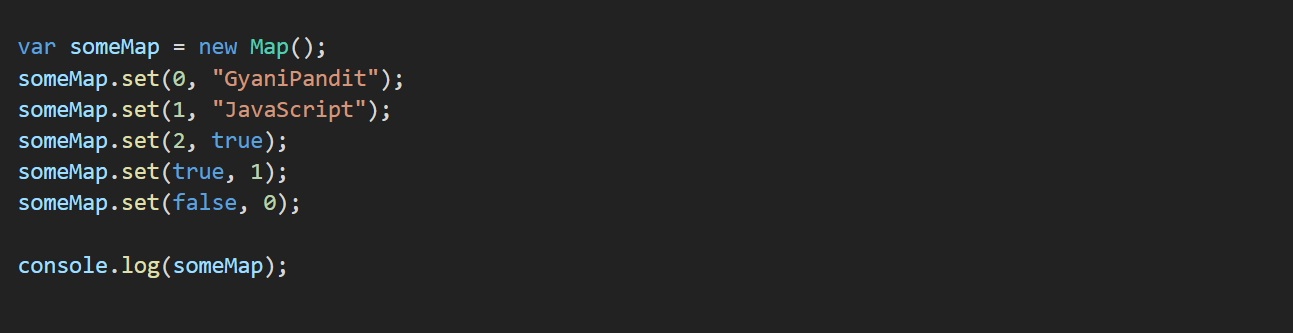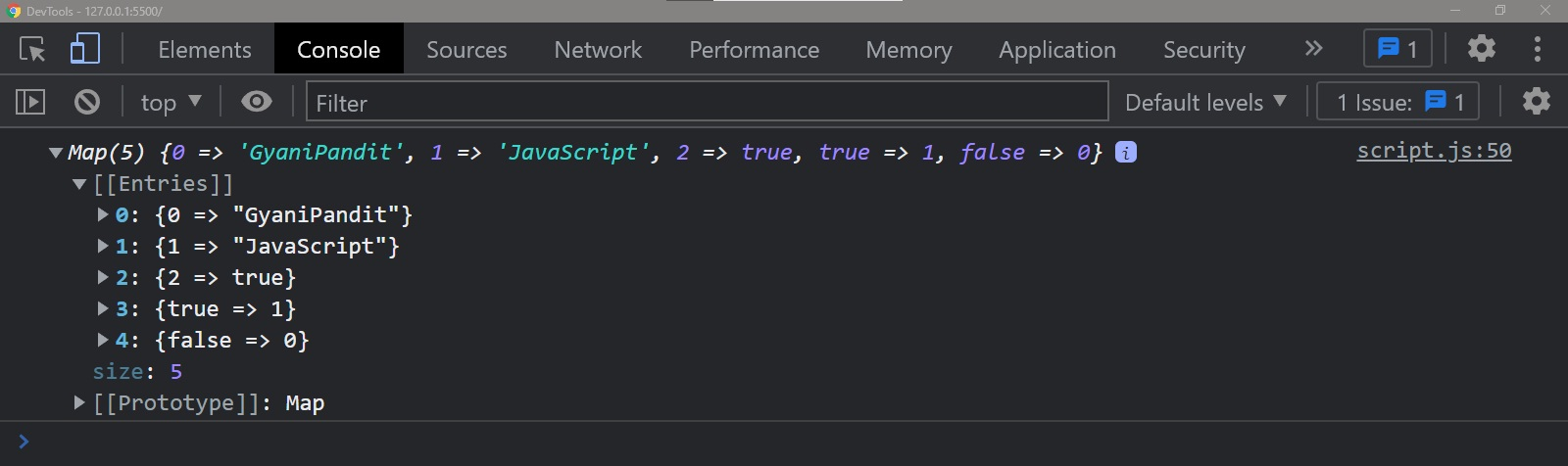# JavaScript Map set() Method

Setting the key-value pairs in the Map (set method)

Now, we know how to create the Map object, and we have created one, but how about adding some key-value pairs to the Map? Well, we can do this, using the set method.

Let’s have a look at the program now, through which, we are going to understand using the set method. You will be able to see that we are passing two values to the set method.

### JavaScript Map Set

Here, the first value is the key, and the other value is the value. Let’s have a look at the program now –You can observe in the above program, that we have created the Map object, and then we are using the set method, to have some key-value pairs in the Map. We are passing two values to the set method, and the first one is the key, and the other one is the value. In the end, we are trying to print the Map object. Let’s have a look at the output now –As you can see, we got the Map object with some key-value pairs here. This was easy.